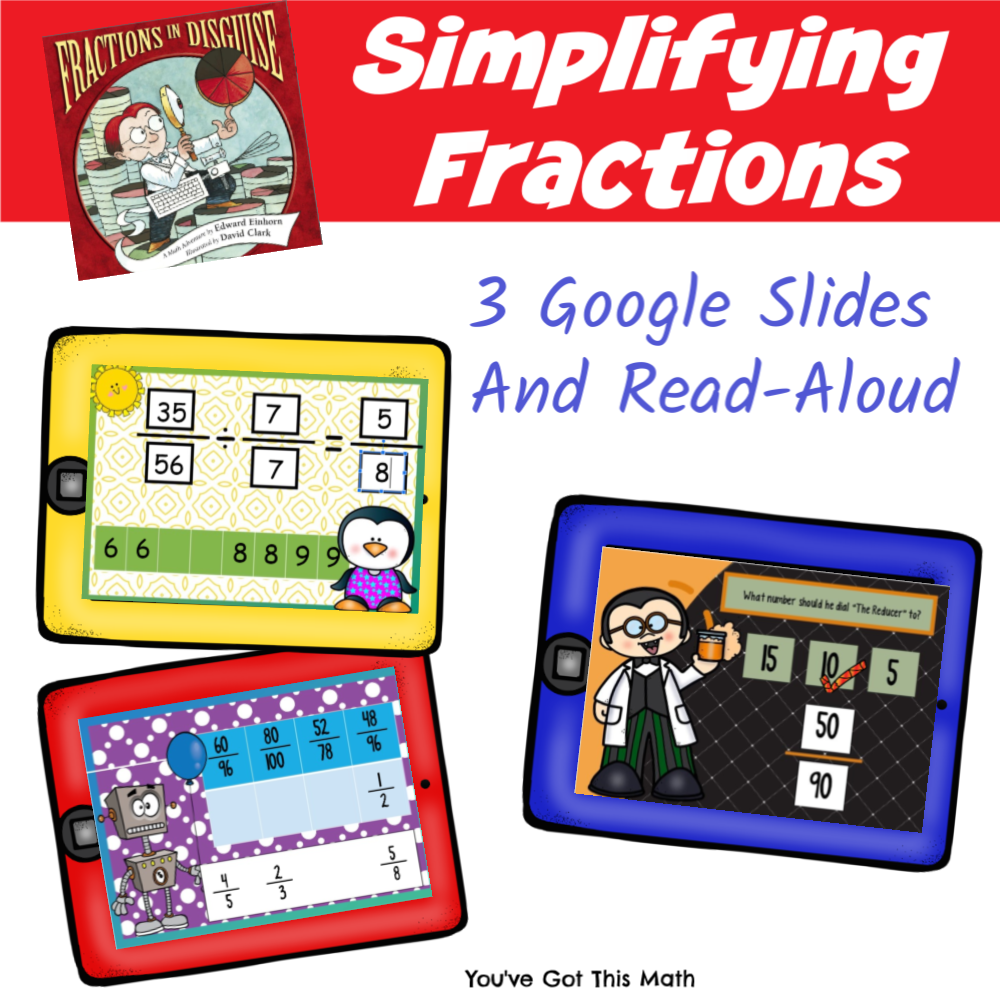# THREE Fun Digital Resources to Work on Simplifying Fractions

Read the delightful book “Fractions in Disguise”, then grab the three simplifying fractions digital resources. What a fun way to introduce the concept of simplifying fractions and practicing it.

## Read – Aloud Time

This activity begins with this delightful book called “Fractions in Disguise”.

Many math books don’t have much of a plot, but this one does. There is a little mystery and danger in it, while the concept of simplifying fractions is introduced.

As always, I recommend you personally reading this book to your children. This way you can stop, and discuss it.

For example, when the boy is creating “the reducer”, he says he dials it to the two and it doesn’t work. Stopping them and showing your children why dividing _____by 2/2 doesn’t work is a great way to begin your lesson on reducing fractions and help them understand the book.

But if you are in a bind, you can watch it being read on YouTube

## Greatest Common Factor

After reading, it is time to jump into our first set of Google Slides.

The first set is a great intro and is a perfect companion to the book.

The students are asked to figure out what number to dial “The Reducer” to. In reality, they are looking for the Greatest Common Factor.

On this slide, the students are given the fraction 12/30 and the need to find the GCF.

To do this they can list the factors of 12 and 30.

12 – 1, 2, 3, 4, 6,12

30 – 1, 2, 3, 5, 6, 10, 15, 30

The greatest common factor and the number we would use to simplify the fraction is the six. On the Google slides, the children will drag and drop the checkmark on the correct GCF.

## Simplifying Fractions

The next digital resource takes us a step further.

Now the children get to drag and drop the GCF into a fraction, and then simplify.

In this example, the children are given the fraction 21/63.

They can begin by listing out the factors.

28 – 1, 2, 4, 7, 14, 28

49 – 1, 7, 49

The GCF is 7, so they will drag and drop the 7’s into the divisor.

Now, all that is left is to divide 28 by 7 which is 4, and 49 by 7 which is 7. The simplified answer is 3/7.

## Simplifying Fractions Sort

We finish this bundle with a sort.

Now the children are given four fractions that need to be simplified. They simplify those fractions, find that fraction, and drag it underneath the equivalent fraction.

This last slide is designed to take away the support they had on the other two slides, and figure out the answer for themselves.

These digital slides are a fun, no-prep way to work through simplifying fractions!!!

You may also like:

Simplifying Fraction BINGO

## Grab your DIGITAL RESOURCES HERE!!!